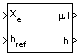# Flat Earth to LLA

Estimate geodetic latitude, longitude, and altitude from flat Earth position

• Library:
• Aerospace Blockset / Utilities / Axes Transformations

•## Description

The Flat Earth to LLA block converts a 3-by-1 vector of flat Earth position$\left(\overline{p}\right)$ into geodetic latitude $\left(\overline{\mu }\right)$, longitude $\left(\overline{\iota }\right)$, and altitude (h). For more information on the flat Earth coordinate system, see Algorithms.

## Limitations

• This estimation method assumes the flight path and bank angle are zero.

• This estimation method assumes the flat Earth z-axis is normal to the Earth at the initial geodetic latitude and longitude only. This method has higher accuracy over small distances from the initial geodetic latitude and longitude, and nearer to the equator. The longitude will have higher accuracy when there are smaller the variations in latitude. Additionally, longitude is singular at the poles.

## Ports

### Input

expand all

Position in flat Earth frame, specified as a 3-by-1 vector.

Data Types: `double`

Reference height from surface of Earth to flat Earth frame with regard to Earth frame, specified as a scalar in the same units as the flat Earth position.

Data Types: `double`

Reference location, specified as a 2-by-1 vector, in degrees of latitude and longitude, for the origin of the estimation and the origin of the flat Earth coordinate system. Use this port if you want to specify the reference location as a dynamic value.

#### Dependencies

This port is enabled if the Input reference position and orientation check box is selected.

Data Types: `double`

Angle, specified as a scalar, for converting flat Earth x and y coordinates to North and East coordinates. Use this port if you want to specify the angle as a dynamic value.

#### Dependencies

This port is enabled if the Input reference position and orientation check box is selected.

Data Types: `double`

### Output

expand all

Geodetic latitude and longitude, returned as a 2-by-1 vector, in degrees.

Data Types: `double`

Altitude above the input reference altitude, returned as a scalar, in the same units as the flat Earth position.

Data Types: `double`

## Parameters

expand all

Parameter and output units.

Units

Position

Altitude

`Metric (MKS)`

Meters

Meters

Meters

`English`

Feet

Feet

Feet

#### Dependencies

To enable this parameter, set Planet model to `Earth (WGS84)`.

#### Programmatic Use

 Block Parameter: `units` Type: character vector Values: ```'Metric (MKS)'``` | `'English'` Default: ```'Metric (MKS)'```

Planet model to use, specified as either `Custom` or `Earth (WGS84)`.

#### Dependencies

Selecting the `Custom` option enables these parameters:

• Flattening

#### Programmatic Use

 Block Parameter: `ptype` Type: character vector Values: ```'Earth (WGS84)'``` | `'Custom'` Default: ```'Earth (WGS84)'```

Flattening of the planet, specified as a double scalar.

#### Dependencies

To enable this parameter, set Planet model to `Custom`.

#### Programmatic Use

 Block Parameter: `F` Type: character vector Values: double scalar Default: `1/298.257223563`

Radius of the planet at its equator, specified as a double scalar, in the same units as the Units parameter.

#### Dependencies

This parameter is enabled when Planet model is set to `Custom`.

#### Programmatic Use

 Block Parameter: `R` Type: character vector Values: double scalar Default: `6378137`

Select this check box to enable ports for reference position and angle to convert flat Earth. Select this check box if you want to specify the reference positions and angle as dynamic values.

#### Dependencies

Selecting this check box replaces these parameters:

• Reference geodetic latitude and longitude [deg]

• Direction of flat Earth x-axis (degrees clockwise from north)

with these input ports:

• μref lref

• ψref input ports.

#### Programmatic Use

 Block Parameter: `refPosPort` Type: character vector Values: `'off'` | `'on'` Default: `'off'`

Reference location in latitude and longitude, specified as 2-by-1 vector, in degrees.

#### Dependencies

To enable this parameter, clear the Input reference position and orientation check box.

#### Programmatic Use

 Block Parameter: `LL0` Type: character vector Values: 2-by-1 vector Default: `[0 10]`

Angle to convert flat Earth x and y coordinates to North and East coordinates, specified as a scalar double, in degrees.

#### Dependencies

This parameter is disabled if the Input reference position and orientation check box is selected.

#### Programmatic Use

 Block Parameter: `psi` Type: character vector Values: double scalar Default: `0`

## Algorithms

The flat Earth coordinate system assumes the z-axis is downward positive. The estimation begins by transforming the flat Earth x and y coordinates to North and East coordinates. The transformation has the form of:

`$\left[\begin{array}{c}N\\ E\end{array}\right]=\left[\begin{array}{cc}\mathrm{cos}\psi & -\mathrm{sin}\psi \\ \mathrm{sin}\psi & \mathrm{cos}\psi \end{array}\right]\left[\begin{array}{c}{p}_{x}\\ {p}_{y}\end{array}\right],$`

where$\left(\overline{\psi }\right)$ is the angle in degrees clockwise between the x-axis and north.

To convert the North and East coordinates to geodetic latitude and longitude, the radius of curvature in the prime vertical (RN) and the radius of curvature in the meridian (RM) are used.

(RN) and (RM) are defined by the following relationships:

`$\begin{array}{l}{R}_{N}=\frac{R}{\sqrt{1-\left(2f-{f}^{2}\right){\mathrm{sin}}^{2}{\mu }_{0}}}\\ {R}_{M}={R}_{N}\frac{1-\left(2f-{f}^{2}\right)}{1-\left(2f-{f}^{2}\right){\mathrm{sin}}^{2}{\mu }_{0}}\end{array}$`

where (R) is the equatorial radius of the planet and$\left(\overline{f}\right)$ is the flattening of the planet.

Small changes in the in latitude and longitude are approximated from small changes in the North and East positions by:

`$\begin{array}{l}d\mu =\text{atan}\left(\frac{1}{{R}_{M}}\right)dN\\ d\iota =\text{atan}\left(\frac{1}{{R}_{N}\mathrm{cos}\mu }\right)dE\end{array}$`

The output latitude and longitude are simply the initial latitude and longitude plus the small changes in latitude and longitude:

`$\begin{array}{l}\mu ={\mu }_{0}+d\mu \\ \iota ={\iota }_{0}+d\iota \end{array}$`

The altitude is the negative flat Earth z-axis value minus the reference height (href):

`$h=-{p}_{z}-{h}_{ref}.$`

 Stevens, B. L., and F. L. Lewis. Aircraft Control and Simulation, Hoboken, NJ: John Wiley & Sons, 2003.

 Etkin, B. Dynamics of Atmospheric Flight Hoboken, NJ: John Wiley & Sons, 1972.

## SupportGet trial now